## A rock is launched at an angle θ = 53.2° above the horizontal from an altitude h = 182 km with an initial speed v0 = ___________________

Question

A rock is launched at an angle θ = 53.2° above the horizontal from an altitude h = 182 km with an initial speed v0 = ____________________ km/s. What

in progress 0
5 months 2021-08-10T14:31:37+00:00 2 Answers 11 views 0

1. Question is incomplete.

Complete question is;

A rock is launched at an angle theta = 53.2 degree above the horizontal from an altitude h = 182 km with an initial speed vo = 1.31 km/s. What is the rock’s speed (m/s) when it reaches an altitude of h/2? (Assume g is a constant 9.8 m/s².)

Rock’s speed = V = 1870.72 m/s

Explanation:

From the question, we are given that;

h = 182km = 182,000m

H = h/2 = 91km = 91000m

Vo = 1.31km/s = 1310m/s

θ = 53.2°

g= 9.8 m/s²

We can find the final speed V at the altitude, H = h/2 by;

H = h + (VoSinθ)t – 1/2×gt²

Where,

t = time taken to reach the height H

Plugging in the relevant values;

91000 = 182000 + (1310Sin53.2°)×t – ((1/2) × 9.8 × t²)

0 = 182000 – 91000 + 1048.96t – 4.9t²

0 = 91000 + 1048.96t – 4.9t²

Thus,

4.90t² – 1048.96t –91000 = 0

By using quadratic equation, we’ll obtain;

t = 280.32 seconds and –66.25 seconds

t cannot be negative and so we pick the positive one.

So, t = 280.32 s

Now, V has both horizontal and vertical components which we can calculate;

The vertical component Vy at t = 280.32s;

Vy = VoSinθ –gt

Vy = 1310sin53.2° – 9.8 × 280.32 = –1698.18 m/s

Now, for the horizontal component, Vx is constant and doesn’t change during the flight of the rock.

Thus, acceleration is zero. So,

Vx = VoCosθ = 1310Cos53.2 = 784.72m/s

Now, the magnitude of the final velocity will be the resultant of Vy and Vx.

Thus,

V = √(Vy² + Vx²)

Plugging in the relevant values,

V =√(-1698.18² + 784.72²)

V =√3499600.7908

V = 1870.72 m/s

A rock is launched at an angle theta = θ = 53.2° above the horizontal from an altitude h = 182 km with an initial speed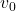= 1.31 km/s. What is the rock’s speed (m/s) when it reaches an altitude of h/2? (Assume g is a constant 9.8 m/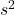)

The speed of the rocks is 1870.74 m/s

Explanation:

The initial potential and kinetic energy of the system is equal to the final kinetic and potential according to the law of conservation of energy.

Initial (PE + KE) = Final (PE + KE)

P E = mgh

K.E = 1/2m

This implies that;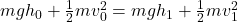…………….1

Where m is the mass;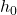is the initial height = 182 km = 182 km x 1000 = 182 000 m

g is acceleration due to gravity = 9.8 m/is the initial velocity = 1.31 km/s = 1.31 km/s x 1000 m/km = 1310 m/s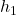is the final height = h/2 = 182000/2 = 91000 m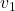is the final velocity;

Rearranging equation 1 to makethe subject formula, we cancel out m;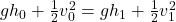Substitution into the equation;

(9.8 x 182000) + (1310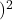/2 = (9.8 x 91000) +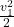1783600 + 858050 = 891800  +2641650 – 891800 == 1749850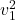= 3499700=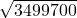= 1870.74 m/s

Therefore the rocks speed is 1870.74 m/s